## ES6 Math方法和Number新特性简介### 一、ES6中的Math对象

#### Math.sign(x)

`Math.sign()`函数返回一个数字的符号, 指示数字是正数，负数还是零。

```Math.sign(3);         //  1
Math.sign(-3);        // -1
Math.sign("-3");      // -1
Math.sign(0);         //  0
Math.sign(-0);        // -0
Math.sign(-Infinity); // -1
Math.sign(Infinity);  //  1
Math.sign(NaN);       // NaN
Math.sign("foo");     // NaN
Math.sign();          // NaN```

```if (!Math.sign) {
Math.sign = function(x) {
// 如果 x 是 NaN, 结果是 NaN.
// 如果 x 是 -0，结果是 -0.
// 如果 x 是 +0，结果是 +0.
// 如果 x 是 负数但不是 -0，结果是 -1.
// 如果 x 是 正数但不是 +0，结果是 +1.
x = +x; // 转换成数值
if (x === 0 || isNaN(x)) {
return Number(x);
}
return x > 0 ? 1 : -1;
};
}```

#### Math.trunc(x)

```Math.trunc(13.37)    // 13
Math.trunc(42.84)    // 42
Math.trunc(0.123)    //  0
Math.trunc(-0.123)   // -0
Math.trunc("-1.123") // -1
Math.trunc(NaN)      // NaN
Math.trunc("foo")    // NaN
Math.trunc()         // NaN```

```if (!Math.trunc) {
Math.trunc = function(v) {
v = +v;
return (v - v % 1) || (!isFinite(v) || v === 0 ? v : v < 0 ? -0 : 0);
};
}```

#### Math.cbrt(x)

`Math.cbrt()`函数返回任意数字的立方根。

```Math.cbrt(8); // 2
Math.cbrt(NaN); // NaN
Math.cbrt(-1); // -1
Math.cbrt(-0); // -0
Math.cbrt(-Infinity); // -Infinity
Math.cbrt(0); // 0
Math.cbrt(1); // 1
Math.cbrt(Infinity); // Infinity
Math.cbrt(null); // 0
Math.cbrt(2);  // 1.2599210498948734```

Polyfill代码如下，可以兼容老旧的浏览器：

```if (!Math.cbrt) {
Math.cbrt = function(x) {
var y = Math.pow(Math.abs(x), 1/3);
return x < 0 ? -y : y;
};
}```

#### Math.expm1(x)

`Math.expm1()` 函数返回 Ex - 1，其中 x 是该函数的参数, E 是自然对数的底数 2.718281828459045。

`Math.expm1()`中的expm1 是 "exponent minus 1" 的缩写，语义上等同于Math.exp(x)-1，但是实际上两者还是有区别的，当Math.exp()的结果接近于1的时候，Math.expm1()的精度更高，例如：

```Math.expm1(1e-10);
// 1.00000000005e-10
Math.exp(1e-10) - 1;
// 1.000000082740371e-10```

`Math.expm1(x)`

```Math.expm1(-1); // -0.6321205588285577
Math.expm1(0);  // 0
Math.expm1(1)     // 1.7182818284590453
Math.expm1(-38)   // -1
Math.expm1("-38") // -1
Math.expm1("foo") // NaN```

Polyfill代码如下，可以兼容Internet Explorer浏览器：

```Math.expm1 = Math.expm1 || function(x) {
return Math.exp(x) - 1;
};```

#### Math.log1p(x)

`Math.log1p()`返回参数值+1的自然对数（e），用数学公式表示就是：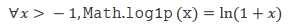```Math.log1p(1);  // 0.6931471805599453
Math.log1p(0);  // 0
Math.log1p(-1); // -Infinity
Math.log1p(-2); // NaN```

JS中使用的双浮点数可以提供大约15位的精度。1+1e-15=1.000000000000001，但1+1e-16=1.000000000000000，因此在该算法中正好是1.0，因为超过15的数字是四舍五入的。

`Math.log1p()`方法IE浏览器不支持，polyfill代码如下：

```Math.log1p = Math.log1p || function(x) {
return Math.log(1 + x);
};```

#### Math.log2(x)

`Math.log2()`方法返回以2为底的对数。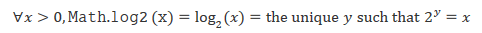```Math.log2(8);    // 3
Math.log2(3);    // 1.584962500721156
Math.log2(2);    // 1
Math.log2(1);    // 0
Math.log2(0);    // -Infinity
Math.log2(-2);   // NaN
Math.log2(1024); // 10```

```if (!Math.log2) Math.log2 = function(x) {
return Math.log(x) * Math.LOG2E;
};```

#### Math.log10(x)

`Math.log10()`方法返回以10为底的对数。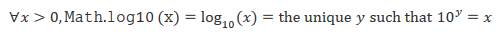```Math.log10(100);    // 2
Math.log10(2);      // 0.3010299956639812
Math.log10(2);      // 0.3010299956639812
Math.log10(1);      // 0
Math.log10(0);      // -Infinity
Math.log10(-2);     // NaN
Math.log10(100000); // 5```

IE浏览器不支持这个Math静态方法，可以使用下面的Polyfill：

```Math.log10 = Math.log10 || function(x) {
return Math.log(x) * Math.LOG10E;
};```

#### Math.fround(x)

`Math.fround()`返回最接近的32位单精度浮点数值。

`var singleFloat = Math.fround(doubleFloat);`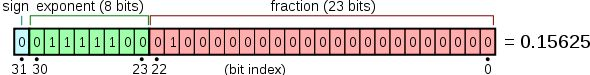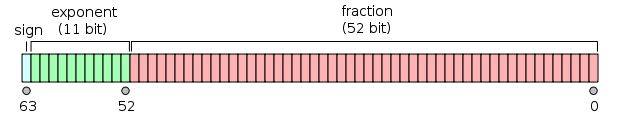```Math.fround(0.8602673475467222);    // 0.8602673411369324
Math.fround(0.8602673499999999);    // 0.8602673411369324
Math.fround(0.8602673400000000);    // 0.8602673411369324
Math.fround(0.86026733);            // 0.8602673411369324```

```Math.fround(1.5);            // 1.5
Math.fround(1.5) === 1.5;    // true```

```Math.fround(1.337);              // 1.3370000123977661
Math.fround(1.337) === 1.337;    // false```

```2 ** 150;                 // 1.42724769270596e+45
Math.fround(2 ** 150);    // Infinity```

```Math.fround('abc');    // NaN
Math.fround(NaN);      // NaN```

`Math.fround()`方法IE浏览器是不认识的，如果项目只需要兼容到IE10+浏览器，则可以使用下面的Polyfill，借助Float32Array对象：

```Math.fround = Math.fround || (function (array) {
return function(x) {
return array = x, array;
};
})(new Float32Array(1));```

```if (!Math.fround) Math.fround = function(arg) {
arg = Number(arg);
// 如果是 ±0 和 NaN 直接返回
if (!arg) return arg;
var sign = arg < 0 ? -1 : 1;
if (sign < 0) arg = -arg;
// 计算指数（8位，有符号）。
var exp = Math.floor(Math.log(arg) / Math.LN2);
var powexp = Math.pow(2, Math.max(-126, Math.min(exp, 127)));
// 处理比较小的值：如果指数位都为零，则前导数字为零。
var leading = exp < -127 ? 0 : 1;
// 尾数23位之后的变成0。
var mantissa = Math.round((leading - arg / powexp) * 0x800000);
if (mantissa <= -0x800000) return sign * Infinity;
return sign * powexp * (leading - mantissa / 0x800000);
};```

#### Math.imul(x, y)

```function idiv(x, y) {
return (x / y) | 0;
}```

```Math.imul(2, 4);          // 8
Math.imul(-1, 8);         // -8
Math.imul(-2, -2);        // 4
Math.imul(0xffffffff, 5); // -5
Math.imul(0xfffffffe, 5); // -10```

IE浏览器的Polyfill如下：

```if (!Math.imul) Math.imul = function(a, b) {
var aHi = (a >>> 16) & 0xffff;
var aLo = a & 0xffff;
var bHi = (b >>> 16) & 0xffff;
var bLo = b & 0xffff;
// 0的移位固定了高位部分的符号
// 最后的| 0将无符号值转换为有符号值
return ((aLo * bLo) + (((aHi * bLo + aLo * bHi) << 16) >>> 0) | 0);
};```

#### Math.clz32(x)

`Math.clz32()`返回参数x的32位二进制表示形式中的前导零位数。

```// 00000000000000000000000000000001
console.log(Math.clz32(1));
// 预期输出: 31

// 00000000000000000000000000000100
console.log(Math.clz32(4));
// 预期输出: 29

// 00000000000000000000001111101000
console.log(Math.clz32(1000));
// 预期输出: 22

Math.clz32(1);           // 31
Math.clz32(1000);        // 22
Math.clz32();            // 32

var stuff = [NaN, Infinity, -Infinity, 0, -0, null, undefined, 'foo', {}, []];
stuff.every(n => Math.clz32(n) == 32);  // true

Math.clz32(true);        // 31
Math.clz32(3.5);         // 30```

```if (!Math.clz32) Math.clz32 = (function(log, LN2){
return function(x) {
var asUint = x >>> 0;
if (asUint === 0) {
return 32;
}
return 31 - (log(asUint) / LN2 | 0) |0; // "| 0" 作用类似于math.floor
};
})(Math.log, Math.LN2);```

#### Math.hypot(...values)

`Math.hypot()`返回参数平方和的平方根。

`Math.hypot([value1[, value2[, ...]]]);`

```Math.hypot(3, 4);        // 5
Math.hypot(3, 4, 5);     // 7.0710678118654755
Math.hypot();            // 0
Math.hypot(NaN);         // NaN
Math.hypot(3, 4, 'foo'); // NaN, +'foo' => NaN
Math.hypot(3, 4, '5');   // 7.0710678118654755, +'5' => 5
Math.hypot(-3);          // 3, the same as Math.abs(-3)```

IE浏览器不支持此方法，可以引入下面的JS代码使之兼容：

```if (!Math.hypot) Math.hypot = function() {
var y = 0, i = arguments.length;
while (i--) y += arguments[i] * arguments[i];
return Math.sqrt(y);
};```

`let distance = Math.hypot(y2-y1, x2-x1);`

#### Math.sinh(x)

`Math.sinh()`返回参数x的双曲正弦值。

```Math.sinh(0);    // 0
Math.sinh(1);    // 1.1752011936438014
Math.sinh(-1);   // -1.1752011936438014
Math.sinh(2);    // 3.626860407847019```

Polyfill代码如下：

```Math.sinh = Math.sinh || function(x) {
return (Math.exp(x) - Math.exp(-x)) / 2;
}```

#### Math.cosh(x)

`Math.cosh()`返回参数x的双曲余弦值。

```Math.cosh(0);    // 1
Math.cosh(1);    // 1.543080634815244
Math.cosh(-1);   // 1.543080634815244
Math.cosh(2);    // 3.7621956910836314```

Polyfill代码如下：

```Math.cosh = Math.cosh || function(x) {
return (Math.exp(x) + Math.exp(-x)) / 2;
}```

#### Math.tanh(x)

`Math.tanh()`返回参数x的双曲正切值。

```Math.tanh(0);        // 0
Math.tanh(Infinity); // 1
Math.tanh(1);        // 0.7615941559557649
Math.tanh(-1);       // -0.7615941559557649```

Polyfill代码如下：

```Math.tanh = Math.tanh || function(x){
var a = Math.exp(+x), b = Math.exp(-x);
return a == Infinity ? 1 : b == Infinity ? -1 : (a - b) / (a + b);
}```

#### Math.asinh(x)

`Math.asinh()`返回参数x的反双曲正弦值。

```Math.asinh(1);    // 0.881373587019543
Math.asinh(0);   // 0
Math.asinh(-1);  // -0.881373587019543
Math.asinh(2)    // 1.4436354751788103```

Polyfill代码如下：

```Math.asinh = Math.asinh || function(x) {
if (x === -Infinity) {
return x;
} else {
return Math.log(x + Math.sqrt(x * x + 1));
}
};```

#### Math.acosh(x)

`Math.acosh()`返回参数x的反双曲余弦值。

```Math.acosh(-1);  // NaN
Math.acosh(0);   // NaN
Math.acosh(0.5); // NaN
Math.acosh(1);   // 0
Math.acosh(2);   // 1.3169578969248166```

Polyfill代码如下：

```Math.acosh = Math.acosh || function(x) {
return Math.log(x + Math.sqrt(x * x - 1));
};```

#### Math.atanh(x)

`Math.atanh()`返回参数x的反双曲正切值。

```Math.atanh(-2);  // NaN
Math.atanh(-1);  // -Infinity
Math.atanh(0);   // 0
Math.atanh(0.5); // 0.5493061443340548
Math.atanh(1);   // Infinity
Math.atanh(2);   // NaN```

Polyfill代码如下：

```Math.atanh = Math.atanh || function(x) {
return Math.log((1+x)/(1-x)) / 2;
};```

### 二、ES6中的Number方法

#### Number.isFinite(number)

`Number.isFinite()`方法用来确定传递的值是否为有限值。

```Number.isFinite(Infinity);  // false
Number.isFinite(NaN);       // false
Number.isFinite(-Infinity); // false

Number.isFinite(0);         // true
Number.isFinite(2e64);      // true

Number.isFinite('0');       // false, 全局的isFinite('0')返回值是true
Number.isFinite(null);      // false, 全局的isFinite(null)返回值是true```

```if (Number.isFinite === undefined) Number.isFinite = function(value) {
return typeof value === 'number' && isFinite(value);
}```

#### Number.isNaN(number)

`Number.isNaN()`方法用来判断参数x是否是NaN，同时类型是Number，这是一个比最初的、全局的`isNaN()`方法更健壮的版本。

```Number.isNaN(NaN);        // true
Number.isNaN(Number.NaN); // true
Number.isNaN(0 / 0);      // true

// 下面这些参数如果使用全局的isNaN()方法都是返回true
Number.isNaN('NaN');      // false
Number.isNaN(undefined);  // false
Number.isNaN({});         // false
Number.isNaN('blabla');   // false

// 下面的全部返回false
Number.isNaN(true);
Number.isNaN(null);
Number.isNaN(37);
Number.isNaN('37');
Number.isNaN('37.37');
Number.isNaN('');
Number.isNaN(' ');```

```Number.isNaN = Number.isNaN || function isNaN(input) {
return typeof input === 'number' && input !== input;
}```

#### Number.parseFloat和Number.parseInt

```Number.parseFloat(string)

Number.parseFloat方法和全局的parseFloat方法是一样的方法。

`Number.parseFloat === parseFloat;    // true`

Number.parseInt方法和全局的parseInt方法是一样的方法。

`Number.parseInt === parseInt;    // true`

#### Number.EPSILON

Number.EPSILON是一个数值很小的常量：

`Number.EPSILON == 2.220446049250313e-16;    // true`

`0.1 + 0.2 === 0.3;    // false`

```function epsEqu(x, y) {
return Math.abs(x - y) < Number.EPSILON;
}
console.log(epsEqu(0.1+0.2, 0.3));   // true```

#### Number.isInteger(number)

JavaScript只有浮点数（双精度）。因此，整数只是不带小数的浮点数。

`Number.isInteger()`方法可以判定数字是不是没有小数，如果没有，则返回`true`

```Number.isInteger(0);         // true
Number.isInteger(1);         // true
Number.isInteger(-100000);   // true
Number.isInteger(99999999999999999999999); // true

Number.isInteger(0.1);       // false
Number.isInteger(Math.PI);   // false

Number.isInteger(NaN);       // false
Number.isInteger(Infinity);  // false
Number.isInteger(-Infinity); // false
Number.isInteger('10');      // false
Number.isInteger(true);      // false
Number.isInteger(false);     // false
Number.isInteger();       // false

Number.isInteger(5.0);       // true
Number.isInteger(5.000000000000001); // false
Number.isInteger(5.0000000000000001); // true```

```Number.isInteger = Number.isInteger || function(value) {
return typeof value === 'number' &&
isFinite(value) &&
Math.floor(value) === value;
};```

#### Safe Integers

• Number.isSafeInteger(number)
• Number.MIN_SAFE_INTEGER
• Number.MAX_SAFE_INTEGER

`Number.isSafeInteger()`方法用来判断参数值是否在安全的整数范围内。

```Number.isSafeInteger(3);                    // true
Number.isSafeInteger(Math.pow(2, 53));      // false
Number.isSafeInteger(Math.pow(2, 53) - 1);  // true
Number.isSafeInteger(NaN);                  // false
Number.isSafeInteger(Infinity);             // false
Number.isSafeInteger('3');                  // false
Number.isSafeInteger(3.1);                  // false
Number.isSafeInteger(3.0);                  // true```

```Number.isSafeInteger = Number.isSafeInteger || function (value) {
return Number.isInteger(value) && Math.abs(value) <= Number.MAX_SAFE_INTEGER;
};```

### 三、ES6中的其它数值变化

ES6中可以使用二进制和八进制表示法指定整数，例如：

```0xFF // ES5: 十六进制

0b11 // ES6: 二进制

0o10 // ES6: 八进制

parseInt()方法可以解析十六进制表示的字符串，例如：

```parseInt('0xFF');         // 255
parseInt('0xFF', 0);      // 255
parseInt('0xFF', 16)      // 255```

```parseInt('0xFF', 10);    // 0
parseInt('0xFF', 17);    // 0```

ES6支持了数值其他表示方法，例如0b11表示3，0o10表示8，但是，`parseInt()`方法在ES6中并没有同步升级，因此，直接`parseInt('0b11')`或者`parseInt('0o10')`不会返回对应的进制值，如下示意：

```parseInt('0b111');       // 0
parseInt('0b111', 2);    // 0
parseInt('111');         // 7

parseInt('0o10');       // 0
parseInt('0o10', 8);    // 0
parseInt('10', 8);      // 8```

```Number('0b111');    // 7
Number('0o10');     // 8```

### 四、结束语（本篇完）

﻿
1. GlacJAY说道：

封面图看不到呀😂️

2. 城北徐工说道：

isInteger 那个可以对 1 取余 === 0

3. 米司特包说道：

学习了

4. foo说道：

+0 == -0 (true), so -0是干什么用的？

5. Ron chan说道：

张鑫旭老师，能请问你这个博客后台用的什么编辑器吗